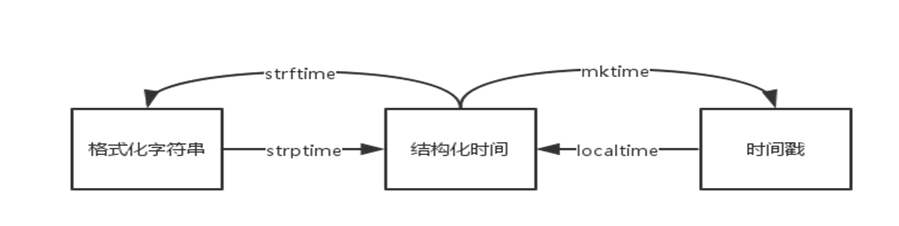# time

1. 时间戳（`timestamp`）：也就是1970年1月1日之后的秒，例如1506388236.216345，可以通过`time.time()`获得。时间戳是一个浮点数，可以进行加减运算，但请注意不要让结果超出取值范围。
2. 格式化的时间字符串（`string_time`）：也就是年月日时分秒这样的我们常见的时间字符串，例如`2017-09-26 09:12:48`，可以通过`time.localtime()`获得;
3. 结构化时间（`struct_time`）：一个包含了年月日时分秒的多元元组，例如`time.struct_time(tm_year=2017, tm_mon=9, tm_mday=26, tm_hour=9, tm_min=14, tm_sec=50, tm_wday=1, tm_yday=269, tm_isdst=0)`，可以通过`time.strftime('%Y-%m-%d')`获得。

UTC（Coordinated Universal Time，世界协调时），亦即格林威治天文时间，世界标准时间。我们中国为东八区，比UTC早8个小时，也就是UTC+8。关于UTC的缩写，有个故事，你可能已经注意到了，按英文的缩写，应该是CUT，而不是UTC。但是世界协调时在法文中的缩写是TUC，两国互相不让，作为妥协，最后干脆简称UTC。

DST（Daylight Saving Time）即夏令时。

## 一、结构化时间（`struct_time`）

```>>> time.localtime()
time.struct_time(tm_year=2017, tm_mon=9, tm_mday=26, tm_hour=10, tm_min=6, tm_sec=49, tm_wday=1, tm_yday=269, tm_isdst=0)
```

0 tm_year（年） 比如2017
1 tm_mon（月） 1 - 12
2 tm_mday（日） 1 - 31
3 tm_hour（时） 0 - 23
4 tm_min（分） 0 - 59
5 tm_sec（秒） 0 - 61
6 tm_wday（weekday） 0 - 6（0表示周一）
7 tm_yday（一年中的第几天） 1 - 366
8 tm_isdst（是否是夏令时） 默认为-1

```>>>import time
>>> lt = time.localtime()
>>> lt
time.struct_time(tm_year=2017, tm_mon=9, tm_mday=26, tm_hour=9, tm_min=27, tm_sec=29, tm_wday=1, tm_yday=269, tm_isdst=0)
>>> lt
9
>>> lt[2:5]
(26, 9, 27)
>>> lt.tm_wday
1
```

```>>> lt.tm_wday = 2
Traceback (most recent call last):
File "<pyshell#12>", line 1, in <module>
lt.tm_wday = 2
```

## 二、 格式化时间字符串

```>>> time.strftime('%Y-%m-%d %H:%M:%S')
'2017-09-26 10:04:28'
```

%a 本地星期名称的简写（如星期四为Thu）
%A 本地星期名称的全称（如星期四为Thursday）
%b 本地月份名称的简写（如八月份为agu）
%B 本地月份名称的全称（如八月份为august）
%c 本地相应的日期和时间的字符串表示（如：15/08/27 10:20:06）
%d 一个月中的第几天（01 - 31）
%f 微秒（范围0.999999）
%H 一天中的第几个小时（24小时制，00 - 23）
%I 第几个小时（12小时制，0 - 11）
%j 一年中的第几天（001 - 366）
%m 月份（01 - 12）
%M 分钟数（00 - 59）
%p 本地am或者pm的标识符
%S 秒（00 - 61）
%U 一年中的星期数。（00 - 53星期天是一个星期的开始。）第一个星期天之 前的所有天数都放在第0周。
%w 一个星期中的第几天（0 - 6，0是星期天）
%W 和%U基本相同，不同的是%W以星期一为一个星期的开始。
%x 本地相应日期字符串（如15/08/01）
%X 本地相应时间字符串（如08:08:10）
%y 去掉世纪的年份（00 - 99）两个数字表示的年份
%Y 完整的年份（4个数字表示年份）
%z 与UTC时间的间隔（如果是本地时间，返回空字符串）
%Z 时区的名字（如果是本地时间，返回空字符串）
%% ‘%’字符

## 三、 time模块主要方法

1. time.sleep(t)

time模块最常用的方法之一，用来睡眠或者暂停程序t秒，t可以是浮点数或整数。

2. time.time()

```>>> time.time()
1506391907.020303
```

```import time

def func():
time.sleep(1.14)
pass

t1 = time.time()
func()
t2 = time.time()
print(t2 - t1)

#print(t2 + 100)
#print(t1 - 10)
#print(t1*2)
```

3. time.gmtime([secs])

```>>> time.gmtime()
time.struct_time(tm_year=2017, tm_mon=9, tm_mday=26, tm_hour=2, tm_min=14, tm_sec=17, tm_wday=1, tm_yday=269, tm_isdst=0)
>>> t = time.time() - 10000
>>> time.gmtime(t)
time.struct_time(tm_year=2017, tm_mon=9, tm_mday=25, tm_hour=23, tm_min=31, tm_sec=3, tm_wday=0, tm_yday=268, tm_isdst=0)
```

4. time.localtime([secs])

```>>> time.localtime()
time.struct_time(tm_year=2017, tm_mon=9, tm_mday=26, tm_hour=10, tm_min=20, tm_sec=42, tm_wday=1, tm_yday=269, tm_isdst=0)
>>> time.localtime(1406391907)
time.struct_time(tm_year=2014, tm_mon=7, tm_mday=27, tm_hour=0, tm_min=25, tm_sec=7, tm_wday=6, tm_yday=208, tm_isdst=0)
>>> time.localtime(time.time() + 10000)
time.struct_time(tm_year=2017, tm_mon=9, tm_mday=26, tm_hour=13, tm_min=7, tm_sec=54, tm_wday=1, tm_yday=269, tm_isdst=0)
```

5. time.ctime([secs])

```>>> time.ctime()
'Tue Sep 26 10:22:31 2017'
>>> time.ctime(time.time())
'Tue Sep 26 10:23:51 2017'
>>> time.ctime(1406391907)
'Sun Jul 27 00:25:07 2014'
>>> time.ctime(time.time() + 10000)
'Tue Sep 26 13:11:55 2017'
```

6. time.asctime([t])

```>>> time.asctime()
'Tue Sep 26 10:27:23 2017'
>>> time.asctime(time.time())
Traceback (most recent call last):
File "<pyshell#13>", line 1, in <module>
time.asctime(time.time())
TypeError: Tuple or struct_time argument required
>>> time.asctime(time.localtime())
'Tue Sep 26 10:27:45 2017'
>>> time.asctime(time.gmtime())
'Tue Sep 26 02:27:57 2017'
```

7. time.mktime(t)

```>>> time.mktime(1406391907)
Traceback (most recent call last):
File "<pyshell#16>", line 1, in <module>
time.mktime(1406391907)
TypeError: Tuple or struct_time argument required
>>> time.mktime(time.localtime())
1506393039.0
```

8. time.strftime(format [, t])

```>>> time.strftime("%Y-%m-%d %H:%M:%S")
'2017-09-26 10:34:50'
>>> time.strftime("%Y-%m-%d %H:%M:%S",time.gmtime())
'2017-09-26 02:34:53'
```

9. time.strptime(string[,format])

```>>> import time
>>> stime = "2017-09-26 12:11:30"
>>> st  = time.strptime(stime,"%Y-%m-%d %H:%M:%S")
>>> st
time.struct_time(tm_year=2017, tm_mon=9, tm_mday=26, tm_hour=12, tm_min=11, tm_sec=30, tm_wday=1, tm_yday=269, tm_isdst=-1)
>>> for item in st:
print(item)

2017
9
26
12
11
30
1
269
-1
>>> wrong_time = "2017-14-26 12:11:30"
>>> st  = time.strptime(wrong_time,"%Y-%m-%d %H:%M:%S")
Traceback (most recent call last):
File "<pyshell#8>", line 1, in <module>
st  = time.strptime(wrong_time,"%Y-%m-%d %H:%M:%S")
File "C:\Python36\lib\_strptime.py", line 559, in _strptime_time
tt = _strptime(data_string, format)
File "C:\Python36\lib\_strptime.py", line 362, in _strptime
(data_string, format))
ValueError: time data '2017-14-26 12:11:30' does not match format '%Y-%m-%d %H:%M:%S'
```

10. time.clock()

```import time

def procedure() :
time.sleep(3)

time1 = time.clock()
procedure()
print(time.clock() - time1, "seconds process time!")
```

```2.999257758349577 seconds process time!
```

## 四、 时间格式之间的转换

Python的三种类型时间格式，可以互相进行转换，如下图和下表所示：UTC结构化时间 时间戳 calendar.timegm()

```>>> t = time.time()         # t是一个时间戳
>>> time.gmtime(t - 10000)      # t减去1万秒，然后转换成UTC结构化时间
time.struct_time(tm_year=2017, tm_mon=9, tm_mday=25, tm_hour=22, tm_min=50, tm_sec=36, tm_wday=0, tm_yday=268, tm_isdst=0)
>>> lt = time.localtime(t - 10000)  # t减去1万秒，然后转换成中国本地结构化时间
time.struct_time(tm_year=2017, tm_mon=9, tm_mday=26, tm_hour=6, tm_min=50, tm_sec=36, tm_wday=1, tm_yday=269, tm_isdst=0)
>>> time.mktime(lt)     # 从本地结构化时间转换为时间戳
1506379836.0
>>> st = time.strftime("%Y-%m-%d %H:%M:%S",lt)  # 从本地结构化时间转换为时间字符串
>>> st
'2017-09-26 06:50:36'
>>> lt2 = time.strptime(st, "%Y-%m-%d %H:%M:%S") # 从时间字符串转换为结构化时间
>>> lt2
time.struct_time(tm_year=2017, tm_mon=9, tm_mday=26, tm_hour=6, tm_min=50, tm_sec=36, tm_wday=1, tm_yday=269, tm_isdst=-1)
```

#### 评论总数： 2DeprecationWarning: time.clock has been deprecated in Python 3.3 and will be removed from Python 3.8: use time.perf_counter or time.process_time instead